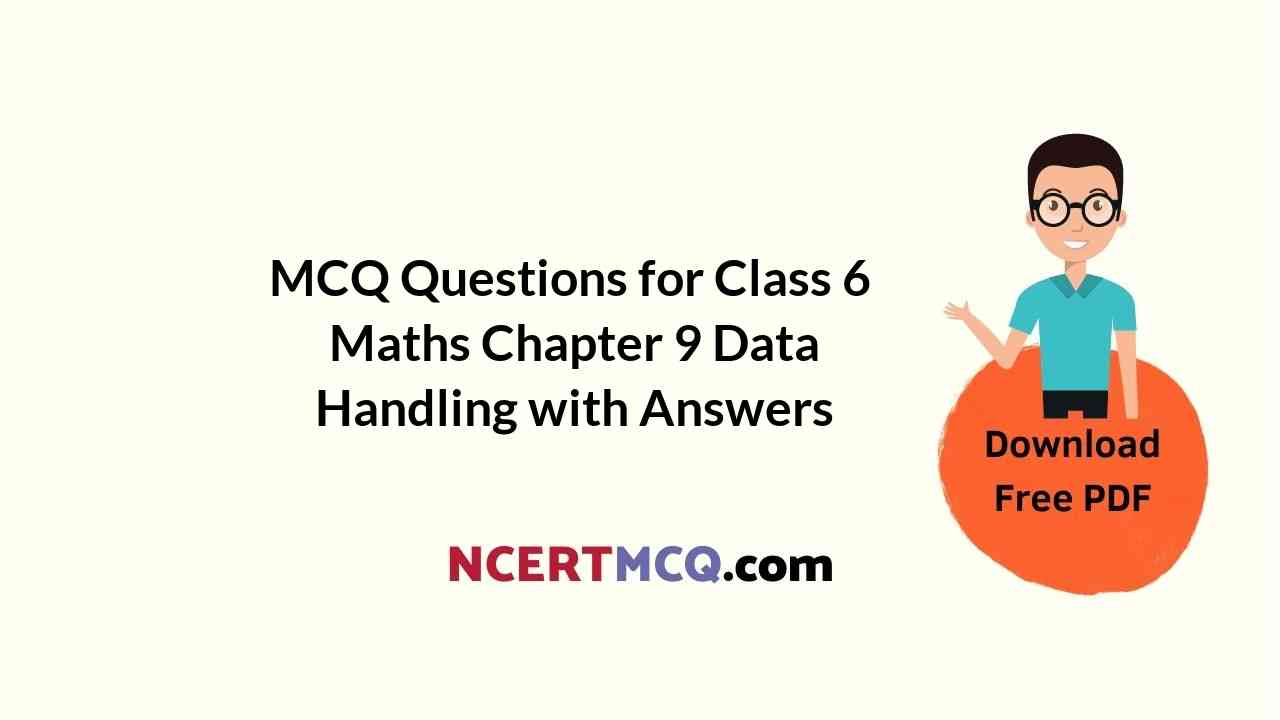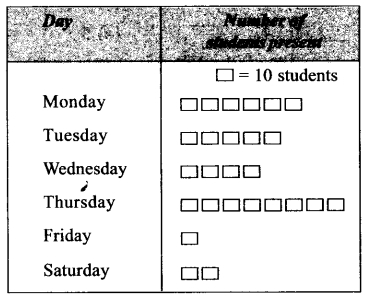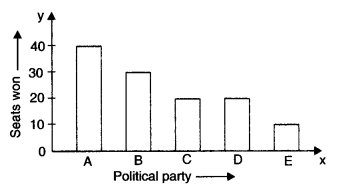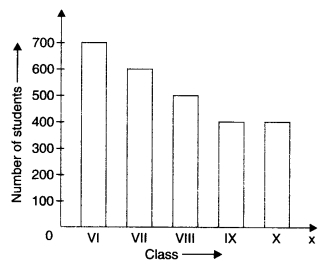Check the below Online Education NCERT MCQ Questions for Class 6 Maths Chapter 9 Data Handling with Answers Pdf free download. MCQ Questions for Class 6 Maths with Answers were prepared based on the latest exam pattern. We have provided Data Handling Class 6 Maths MCQs Questions with Answers to help students understand the concept very well.

Students can also refer to NCERT Solutions for Class 6 Maths Chapter 9 Data Handling for better exam preparation and score more marks.

## Online Education for Data Handling Class 6 MCQs Questions with Answers

Data Handling Class 6 MCQ Question 1.
The maximum marks obtained by any student is
(a) 95
(b) 78
(c) 75
(d) 25.

MCQ On Data Handling For Class 6 Question 2.
The minimum marks obtained by any student is
(a) 95
(b) 78
(c) 75
(d) 25.

Class 6 Maths Chapter 9 MCQ Question 3.
How many students got the same marks?
(a) 2
(b) 3
(c) 4
(d) 5.

Hint:
62, 62

Data Handling MCQ Class 6 Question 4.
The difference between the maximum and mini¬mum marks obtained is
(a) 60
(b) 50
(c) 70
(d) 80.

Hint:
95 – 25 = 70

Class 6 Data Handling MCQ Question 5.
How many students got 75 or more marks?
(a) 1
(b) 2
(c) 3
(d) 4

Hint:
95, 78, 75

MCQ Questions For Class 6 Maths Chapter 9 Question 6.
How many students got marks below 60?
(a) 1
(b) 2
(c) 3
(d) 4.

Hint:
55, 36, 42, 25

MCQ Of Data Handling Class 6 Question 7.
How many students got marks between 60 and 75?
(a) 1
(b) 2
(c) 3
(d) 4.

Hint:
62,62,73

Observe the following table and answer the related questions:Data Handling Class 6 MCQ Questions Question 8.
Which blood group is the most common?
(a) A
(b) B
(c) O
(d) AB.

Class 6 Maths Data Handling MCQ Question 9.
Which blood group is the rarest?
(a) AE
(b) B
(c) A
(d) O.

Ncert Class 6 Maths Chapter 9 MCQ Question 10.
What is the total number of students?
(a) 30
(b) 15
(c) 20
(d) 10.

MCQ Data Handling Class 6 Question 11.
The maximum frequency is
(a) 12
(b) 9
(c) 6
(d) 3.

MCQs On Data Handling Class 6 Question 12.
The minimum frequency is
(a) 3
(b) 6
(c) 9
(d) 12

Class 6 Maths Ch 9 MCQ Question 13.
The difference between maximum and mini-mum frequency is
(a) 3
(b) 6
(c) 9
(d) 12.

Hint:
12 – 3 = 9

MCQ Questions On Data Handling For Class 6 Question 14.
The ratio of the frequencies of blood groups AB and B is
(a) 1:2
(b) 1:3
(c) 2:3
(d) 3:4.

Hint:
3 : 6 = 1 : 2

MCQ Class 6 Maths Chapter 9 Question 15.
The ratio of the frequencies of blood groups B and O is
(a) 1:3
(b) 2:3
(c) 3 :4
(d) 1:2.

Hint:
6 : 12 = 1 : 2

Observe the following pictograph and answer the related questions :Class 6 Math Chapter 9 MCQ Question 16.
On which day were the maximum number of students present?
(a) Monday
(b) Thursday
(c) Tuesday
(d) Saturday.

Data Handling MCQs Class 6 Question 17.
On which day were the minimum number of students present?
(a) Friday
(b) Saturday
(c) Thursday
(d) Wednesday.

MCQ Questions For Class 6 Data Handling Question 18.
The number of students present on Friday is
(a) 10
(b) 20
(c) 40
(d) 50.

MCQ Questions For Class 6 Maths Data Handling Question 19.
On how many days were more than 20 stu-dents present?
(a) 1
(b) 2
(c) 3
(d) 4.

Hint:
Monday, Tuesday, Wednesday, Thursday

MCQ On Bar Graph Class 6 Question 20.
On how many days were less than 20 students present?
(a) 1
(b) 2
(c) 3
(d) 4.

Hint:
Friday

Observe the following bar graph and answer the related questions:Question 21.
Which political party won the maximum number of seats?
(a) A
(b) B
(c) C
(d) D.

Question 22.
Which political party won the minimum number of seats?
(a) A
(b) B
(c) D
(d) E.

Question 23.
Which two political parties won the same number of seats?
(a) C,D
(b) C, E
(c) D, E
(d) B, D.

Hint:
C → 20, D → 20

Question 24.
What is the difference between the maximum and minimum numbers of seats won?
(a) 10
(b) 20
(c) 30
(d) 40.

Hint:
40 – 10 = 30

Question 25.
How many parties won more than 20 seats?
(a) 1
(b) 2
(c) 3
(d) 4.

Hint:
A,B

Question 26.
How many parties won less than 60 seats?
(a) 2
(b) 3
(c) 5
(d) 4.

Hint:
A, B, C, D, E

Question 27.
What is the total number of seats won by party C and party D?
(a) 10
(b) 20
(c) 30
(d) 40.

Hint:
20 + 20 = 40

Observe the following bar graph and answer the related questions:Question 28.
In which class is the number of students maxi¬mum?
(a) VI
(b) VIII
(c) IX
(d) X.

Question 29.
In which class/classes is the number of students minimum?
(a) IX,X
(b) VIII
(c) VI
(d) VII.

Question 30.
In which classes is the number of students are same?
(a) IX,X
(b) VI,VII
(c) VIII,IX
(d) VI,X.

Hint:
IX → 400
X → 400

Question 31.
In which class is the number of students 500?
(i) VIII
(ii) VII
(c) IX
(d) X.

Hint:
500 → VIII

Question 32.
What is the difference between the maximum and the minimum number of students?
(a) 100
(b) 200
(c) 300
(d) 400.

Hint:
700 – 400 = 300

Question 33.
In how many classes is the number of students are same?
(a) 2
(b) 3
(c) 4
(d) 5.

Hint:
IX, X

Question 34.
What is the difference between the number of students of class VI and class VII?
(a) 100
(b) 200
(c) 300
(d) 400.

Hint:
700 – 600 = 100

Question 35.
What is the total number of students?
(a) 2600
(b) 2000
(c) 3600
(d) 1600.

Hint:
700 + 600 + 500 + 400 + 400 = 2600

Question 36.
What is the ratio of the number of students of class X and class IX ?
(a) 1:1
(b) 1:2
(c) 2:1
(d) 1:4.